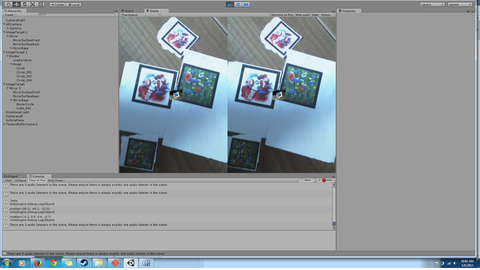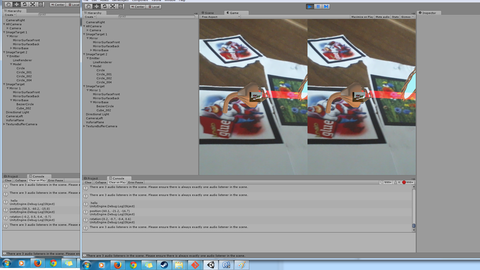# vuforia how to force objects into a 2D plane

I’m working on a game in which cards are placed on a table and then rendered in augmented reality. These objects have 3D physics attached to them which doesn’t work as well if they are either rotated or if the system mistakes them for being higher or lower then they really are.

Is there a good method to force Vuforia/Unity to place all items in the same 2D plane and keep all of them upright compared to that plane?

I currently have the following code:
using UnityEngine;
using System.Collections;

``````public class projectioncode : MonoBehaviour {
Vector3 p1;
Vector3 p2;
Vector3 p3;
Vector3 v1;
Vector3 v2;
Vector3 normal;

/*
*use a plane with the following 3 coordinates
*/
public void setPlaneByPoints(Vector3 point1,Vector3 point2, Vector3 point3){
p1 = point1;
p2 = point2;
p3 = point3;

v1=p2-p1;
v2=p3-p1;
normal=getNormal(v1,v2);
}

public Transform projectTransform(Transform input){
input.position = projectPoint (input.position);
return input;
}
/*
*  projects a 3d point into space;
*/
Vector3 projectPoint(Vector3 point){
Vector3 vOToPoint = point - p1;//vector between origin and point
float dist = Vector3.Dot (vOToPoint, normal); //distance plane to point
return point - dist * normal;
}

/*
* caclulates the normal of 2 vectors
*/
Vector3 getNormal(Vector3 v1,Vector3 v2){
Vector3 res= (Vector3.Cross (v1, v2));
res.Normalize();
return res;
}
}

which seems to kinda project objects into a plane but the results tend to be inaccurate and result in weird movements particular when moving the camera around.
``````Maybe there is also a way to lock movement allong a given axis (note the camera can move).

Man, I want to do the same. I want to make a game with 3D objects moves in a 2D plane to avoid the complexity of doing a true 3D project but still maintain some of the 3D aesthetics. Would appreciate any advice on this topic.